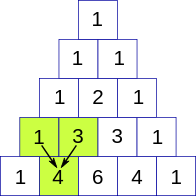New update is available. Click here to update.Close
Topic list
Pascal's Triangle
EASY
20 mins273 upvotes
Matrices (2D Arrays)
Topics (Covered in this problem)
Problem solved
Skill meterMatrices (2D Arrays)
-
-
Other topics
Problem solved
Skill meterStrings
-
--
-Sorting
-
-Binary Search
-
-Stacks & Queues
-
-Trees
-
-Graph
-
-Dynamic Programming
-
-Greedy
-
-Tries
-
-Arrays
-
-SQL
-
-Binary Search Trees
-
-Heap
-
-Bit Manipulation
-
-
Solve problems & track your progress
Checkout your overall progress in every topic here
BecomeSensei
in DSA topics
Open the topic and solve more problems associated with it to improve your skills
Check out the skill meter for every topic
See how many problems you are left with to solve for cracking any stage. Score more than zero to get your progress counted.

# Pascal's Triangle

Contributed by
Dhruv Sharma
Easy0/40
Avg time to solve 20 mins
Success Rate 80 %Share273 upvotes

## Problem Statement

#### A Pascal's triangle is a triangular array constructed by summing adjacent elements in preceding rows. Pascal's triangle contains the values of the binomial coefficient. For example in the figure below.#### For example, given integer N= 4 then you have to print.

``````1
1 1
1 2 1
1 3 3 1

Here for the third row, you will see that the second element is the summation of the above two-row elements i.e. 2=1+1, and similarly for row three 3 = 1+2 and 3 = 1+2.
``````
Detailed explanation ( Input/output format, Notes, Constraints, Images )##### Sample Input 1 :
``````3
1
2
3
``````
##### Sample Output 1 :
``````1
1
1 1
1
1 1
1 2 1
``````
##### Explanation of The Sample Input 1:
``````For the first test case:
The given integer N = 1 you have to print the triangle till row 1 so you just have to output 1.

For the second test case:
The given integer N = 2 you have to print the triangle till row 2 so you have to output
1
1 1

For the third test case
The given integer N = 3 you have to print the triangle till row 3 so you have to output
1
1 1
1 2 1
``````
##### Sample Input 2 :
``````3
4
5
6
``````
##### Sample Output 2 :
``````1
1 1
1 2 1
1 3 3 1
1
1 1
1 2 1
1 3 3 1
1 4 6 4 1
1
1 1
1 2 1
1 3 3 1
1 4 6 4 1
1 5 10 10 5 1
``````AutoConsole